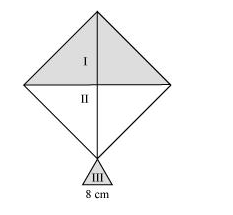# A kite in the shape of a square with a diagonal 32 cmQuestion. A kite in the shape of a square with a diagonal 32 cm and an isosceles triangles of base 8 cm and sides 6 cm each is to be made of three different shades as shown in the given figure. How much paper of each shade has been used in it?Solution:

We know that

Area of square $=\frac{1}{2}$ (diagonal) $^{2}$

Area of the given $k i t e=\frac{1}{2}(32 \mathrm{~cm})^{2}=512 \mathrm{~cm}^{2}$

Area of $1^{\text {st }}$ shade $=$ Area of $2^{\text {nd }}$ shade

$=\frac{512 \mathrm{~cm}^{2}}{2}=256 \mathrm{~cm}^{2}$

Therefore, the area of paper required in each shape is $256 \mathrm{~cm}^{2}$.

For IIIrd triangle

Semi-perimeter, $s=\frac{(6+6+8) \mathrm{cm}}{2}=10 \mathrm{~cm}$

By Heron’s formula,

Area of triangle $=\sqrt{s(s-a)(s-b)(s-c)}$

Area of III $^{\text {rd }}$ triangle $=\sqrt{10(10-6)(10-6)(10-8)}$

$=(\sqrt{10 \times 4 \times 4 \times 2}) \mathrm{cm}^{2}$

$=(4 \times 2 \sqrt{5}) \mathrm{cm}^{2}$

$=8 \sqrt{5} \mathrm{~cm}^{2}$

$=(8 \times 2.24) \mathrm{cm}^{2}$

$=17.92 \mathrm{~cm}^{2}$

Area of paper required for $\| I^{\text {rd }}$ shade $=17.92 \mathrm{~cm}^{2}$